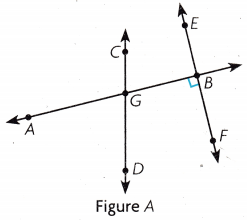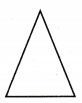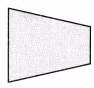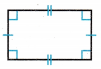Refer to our Texas Go Math Grade 4 Answer Key Pdf to score good marks in the exams. Test yourself by practicing the problems from Texas Go Math Grade 4 Module 13 Assessment Answer Key.

Vocabulary

• acute angle
• line segment
• Obtuse angle
• ray
• right angle
• straight angle

Choose the best term from the box to complete the sentence.

Question 1.
A ____________ is part of a line between two endpoints.
Line segment

Question 2.
A ____________ forms a square corner.
Right angle.

Question 3.
An ____________ is greater than a right angle and less than a straight angle.
Obtuse angle.

Explanation:
Angles larger than a right angle and smaller than a straight angle (between 90° and 180°) are called obtuse angles.

Question 4.
The two-dimensional figure that has one endpoint is a ____________.
Ray

Explanation:
A 2-dimensional figure that has one endpoint and goes without end in one direction. A ray is shown with an endpoint and an arrow at one end.

Question 5.
An angle that forms a line is called a ____________.
Straight angle.

Concepts and Skills

Use Figure A for 6 – 11.Question 6.
Name a ray.
Ray EF

Question 7.
Name an acute angle.
∠AGD

Question 8.
Name a point.
∠B is a point

Question 9.
Name a line segment.
AB is a line segment

Question 10.
Name a pair of perpendicular lines.
EBF is perpendicular to AGB

Question 11.
Name an obtuse angle.
∠AGC is an obtuse angle.

Explanation:
By observing from the given figure the ∠AGC is an obtuse angle that measures more than 90 degrees.

Classify each triangle. Write acute, right or obtuse.

Question 12.Obtuse angle

Explanation:
Obtuse angles measure more than 90 degrees.

Question 13.Right angle

Explanation:
A right angle measures 90 degrees.

Question 14.Acute angle.

Explanation:
The angle measures less than 90 degrees.

Question 15.
Which describes the figure?(A) zero lines of symmetry
(B) 1 line of symmetry
(C) 2 lines of symmetry
(D) more than 2 lines of symmetry
1 line of symmetry

Question 16.
Which figure has 2 pairs of sides that appear to be parallel?
(A)(B)(C)(D)Option A

Question 17.
Which quadrilateral can have 2 pairs of parallel sides, all side with equal length, and no right angles?
(A) square
(B) rhombus
(C) rectangle
(D) trapezoid
Rhombus

Explanation:
A parallelogram with 4 sides with equal length and no right angles is a rhombus.

Question 18.
Which names the figure correctly?(A) line FE
(B) ray FE
(C) angle FE
(D) ray EF